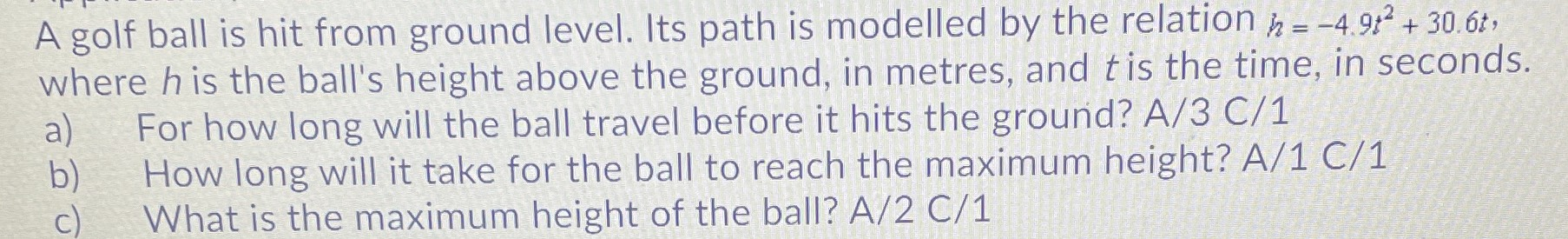### ¿Todavía tienes preguntas de matemáticas?

Pregunte a nuestros tutores expertos
Algebra
PreguntaA golf ball is hit from ground level. Its path is modelled by the relation $$h = - 4.9 t ^ { 2 } + 30.6 t$$ , where $$h$$ is the ball's height above the ground, in metres, and $$t$$ is the time, in seconds.

a) For how long will the ball travel before it hits the ground? $$A / 3 C / 1$$

b) How long will it take for the ball to reach the maximum height? $$A / 1 C / 1$$

c) What is the maximum height of the ball? $$A / 2 C / 1$$